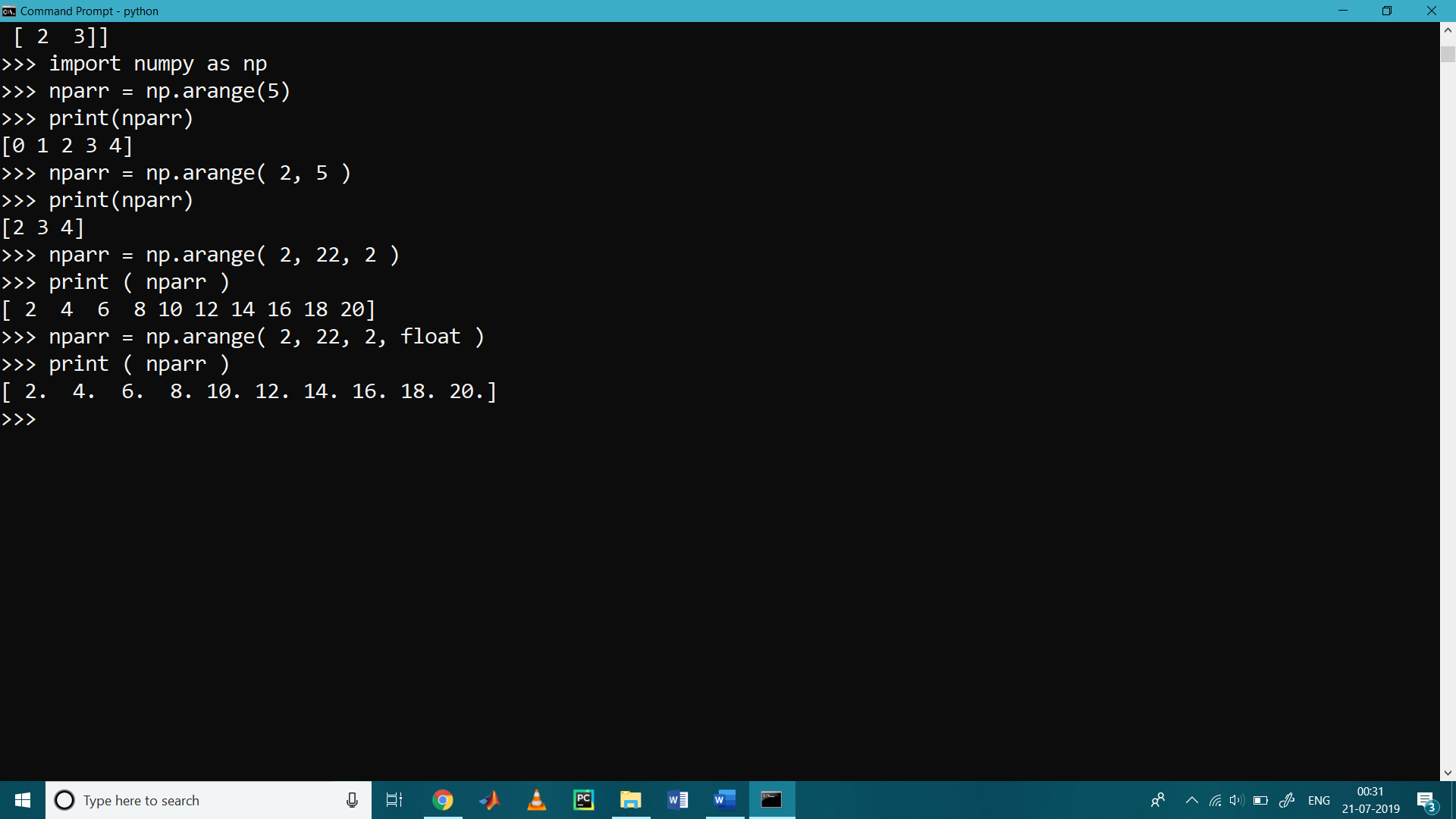Array with Python Numpy

• NumPy is a module for Python. The name is an acronym for “Numeric Python” or “Numerical Python”.
• It is an extension module for Python, mostly written in C.
• It is a successor for two earlier computing Python libraries such as Numeric and numarray.
• It is the fundamental package for scientific computing.
• It is a general-purpose array-processing or manipulating python package.
• It provides a high-level performance on multidimensional array object and tools for working with these arrays.
• It is used for implementing multi-dimensional arrays and matrices.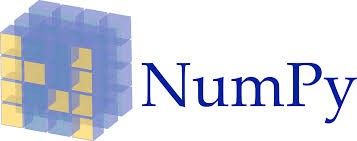Sub Libraries include in NumPy:

• It contains basic linear algebra functions.
• It contains basic Fourier transforms.
• It contains a sophisticated random number of capabilities.
• It contains tools for integrating Fortran code and C/C++ code.
• It contains sophisticated random number capabilities

Application of NumPy:

NumPy is used in various applications such as

• Matrix Computations
• Numerical Analysis
• Image processing
• Signal processing
• Linear algebra
• A plethora of others

Install NumPy

Steps are as follows

Step 1: Open command prompt

Step 2: write pip install numpy

Import NumPy with python

Before we can use NumPy we will have to import it.

>>> import numpy

as it is complicated to write it every time we renamed it as np with the help of following the line of code

>>> import numpy as np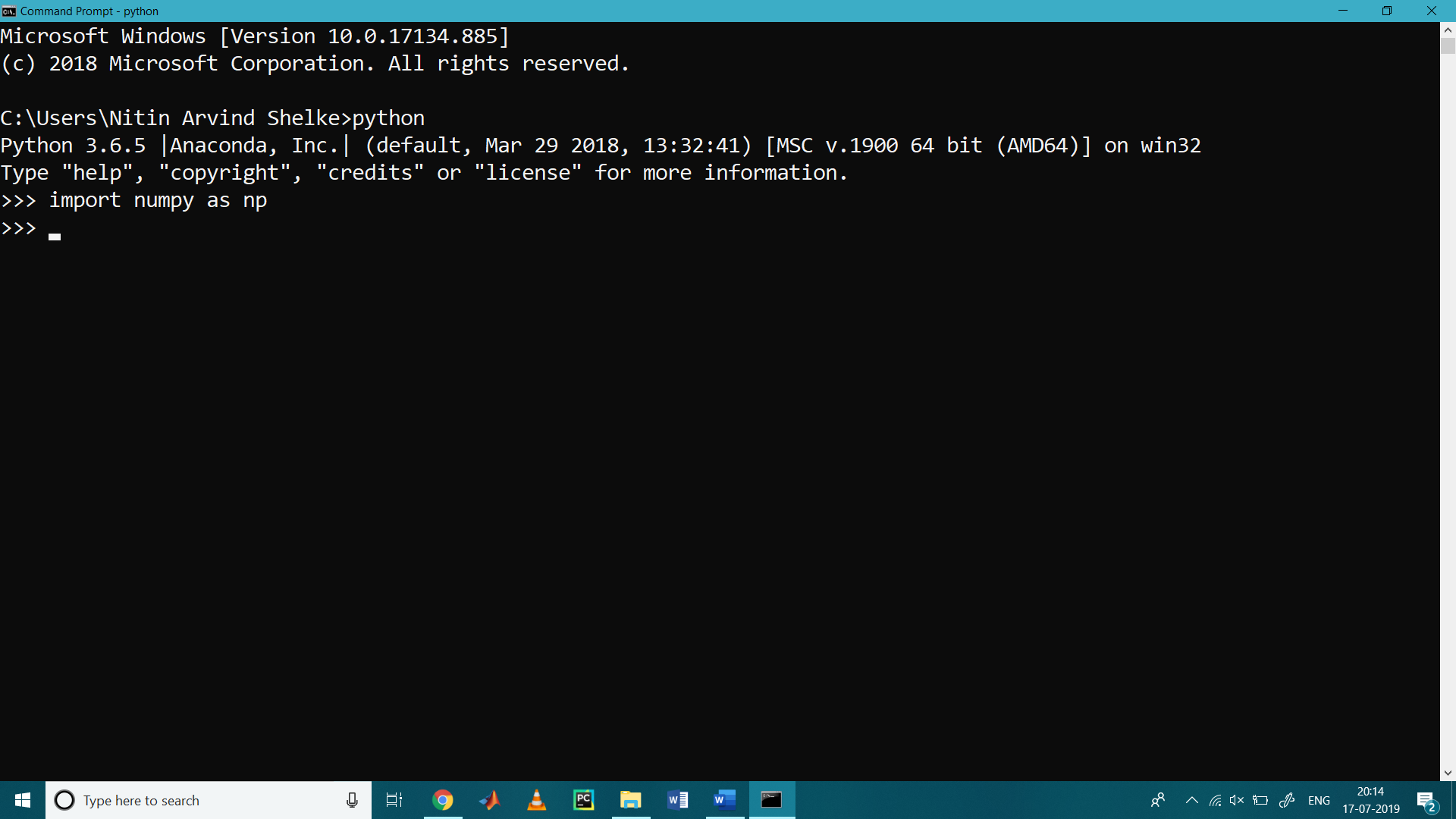Numpy Array

A numpy array is a collection of homogeneous values, all of the same data type, and is indexed by a tuple of nonnegative integers.

The number of dimensions (means count of rows) is the rank of the array.

The shape of an array is a tuple of integers giving the size of the array along each dimension.

Creation of Numpy Array

We can initialize it arrays from nested Python lists, and access elements using square brackets:

Array can be crated with numpy.array

#Creation of 1-D arry

>>> import numpy as np

>>> arr = np.array([8, 9, 10])   # Create a rank 1 array

>>> print(arr)

[ 8  9 10]

>>> type(arr)

<class ‘numpy.ndarray’>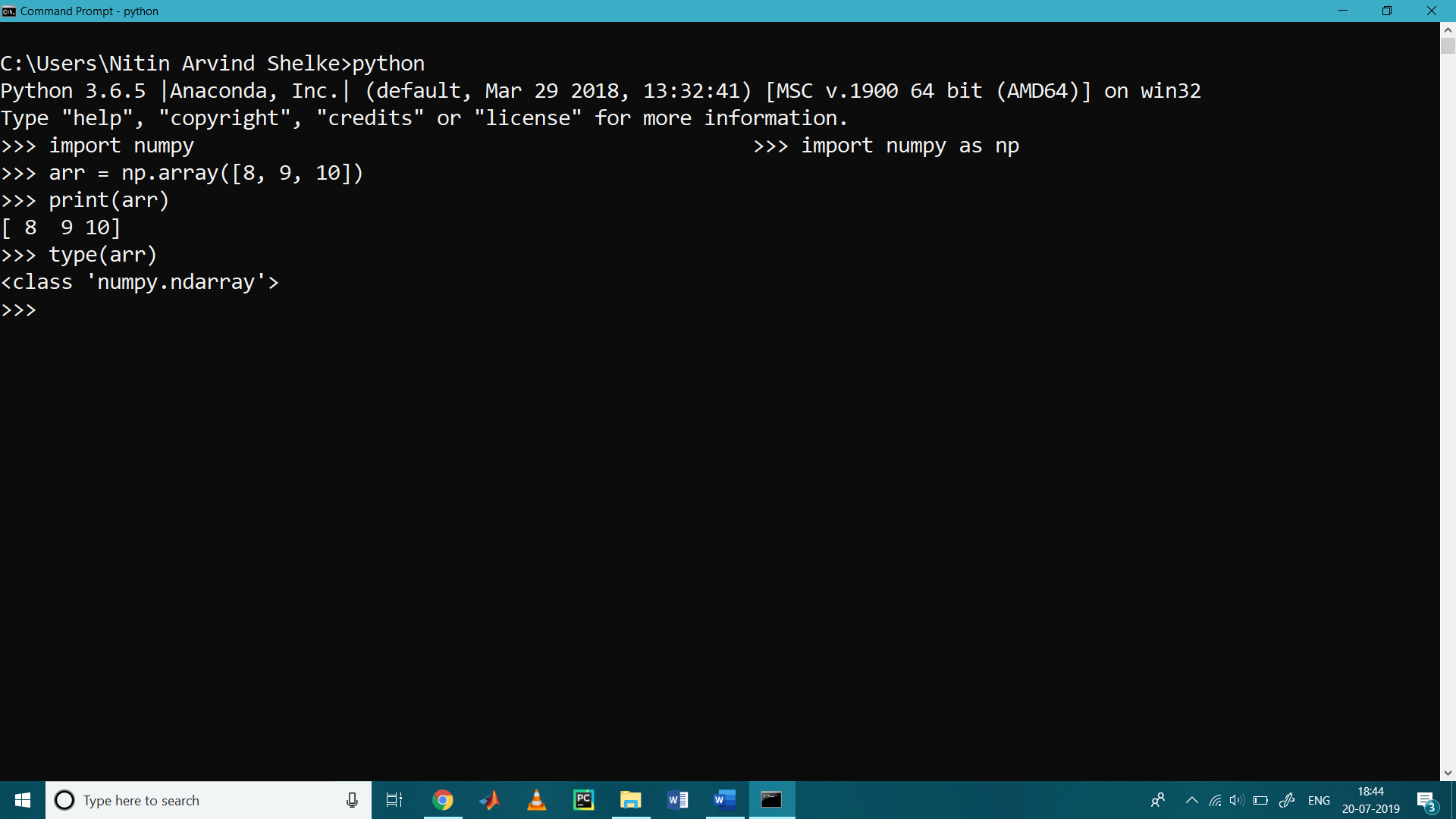Array indexing

It is used to access the particular element of the array

>>> arr = np.array ( [8, 9, 10] )

>>> print ( arr )

8

>>> print ( arr )

9

>>> print ( arr )

10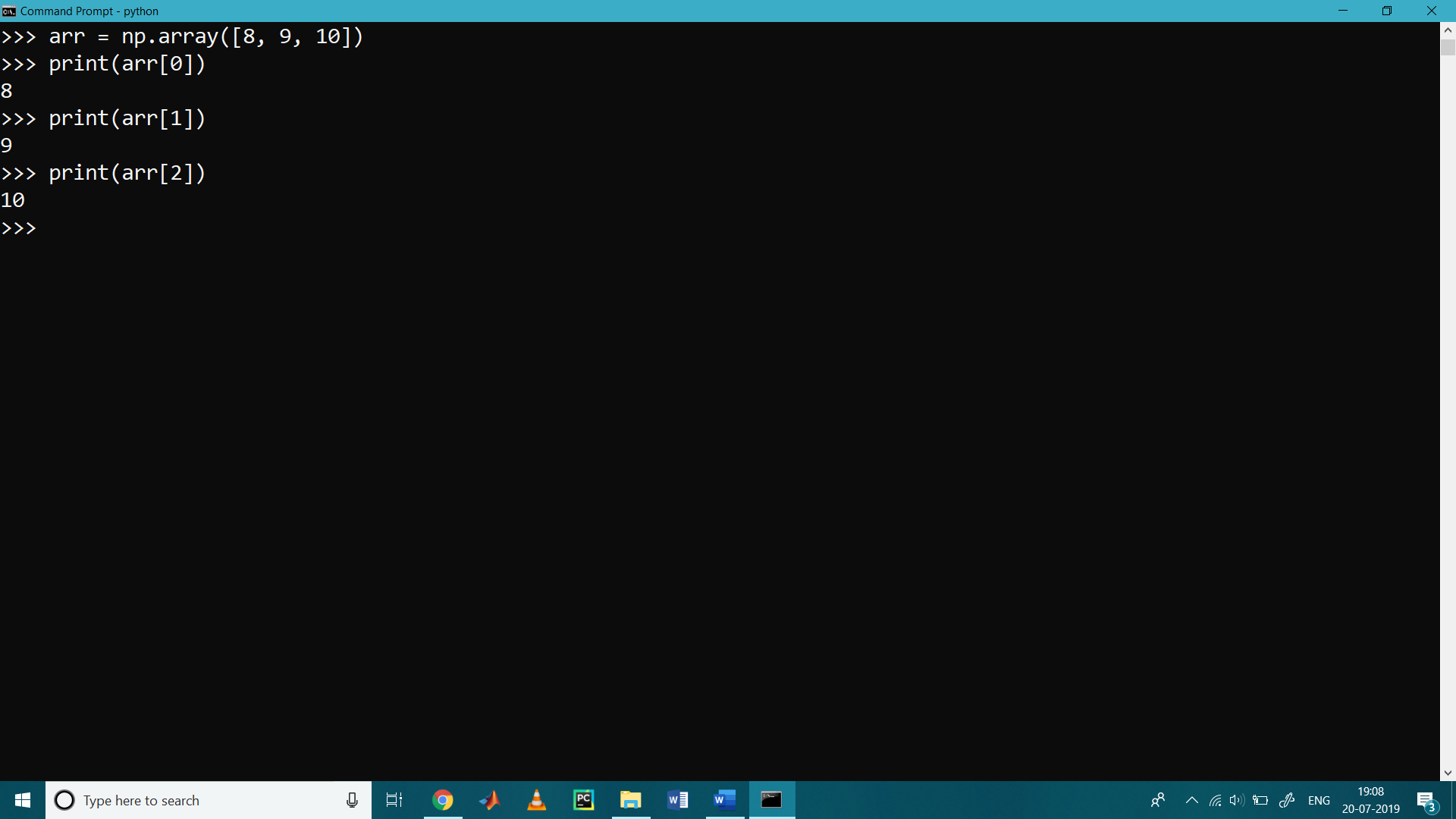To check the array dimension

>>> arr.ndim

1

Shape of the Array

You should check the shape of an array with the object shape preceded by the name of the array.

>>> arr.shape

>>> arr.shape

(3,)

>>>

For a 1D array, the shape would be (n,) where n is the number of elements in your array.

Length of the array

len ( ) method is used to find the length of the array

>>> arr = np.array([8, 9, 10])

>>> print ( len ( arr ) )

3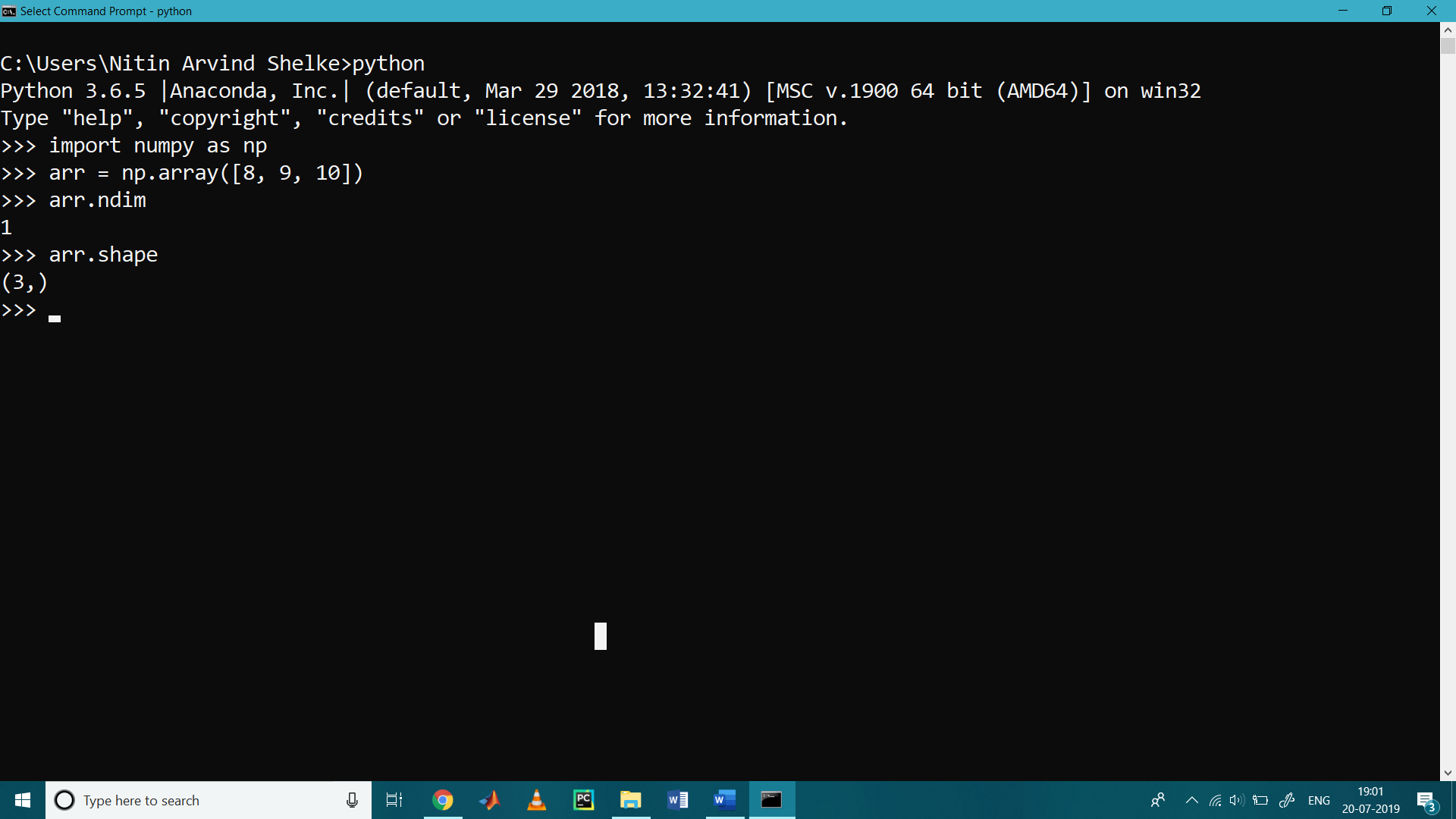Creation of 2-D arry

>>> arr2d = np.array([[0, 1, 2], [3, 4, 5]])

# 2 x 3 array or create a rank of 2

>>> print (arr2d)

[[0 1 2]

[3 4 5]]

>>> print (arr2d.ndim)

2

>>> print (arr2d.shape)

(2, 3)

For a 2D array, the shape would be (n,m) where n is the number of rows and m is the number of columns in your array.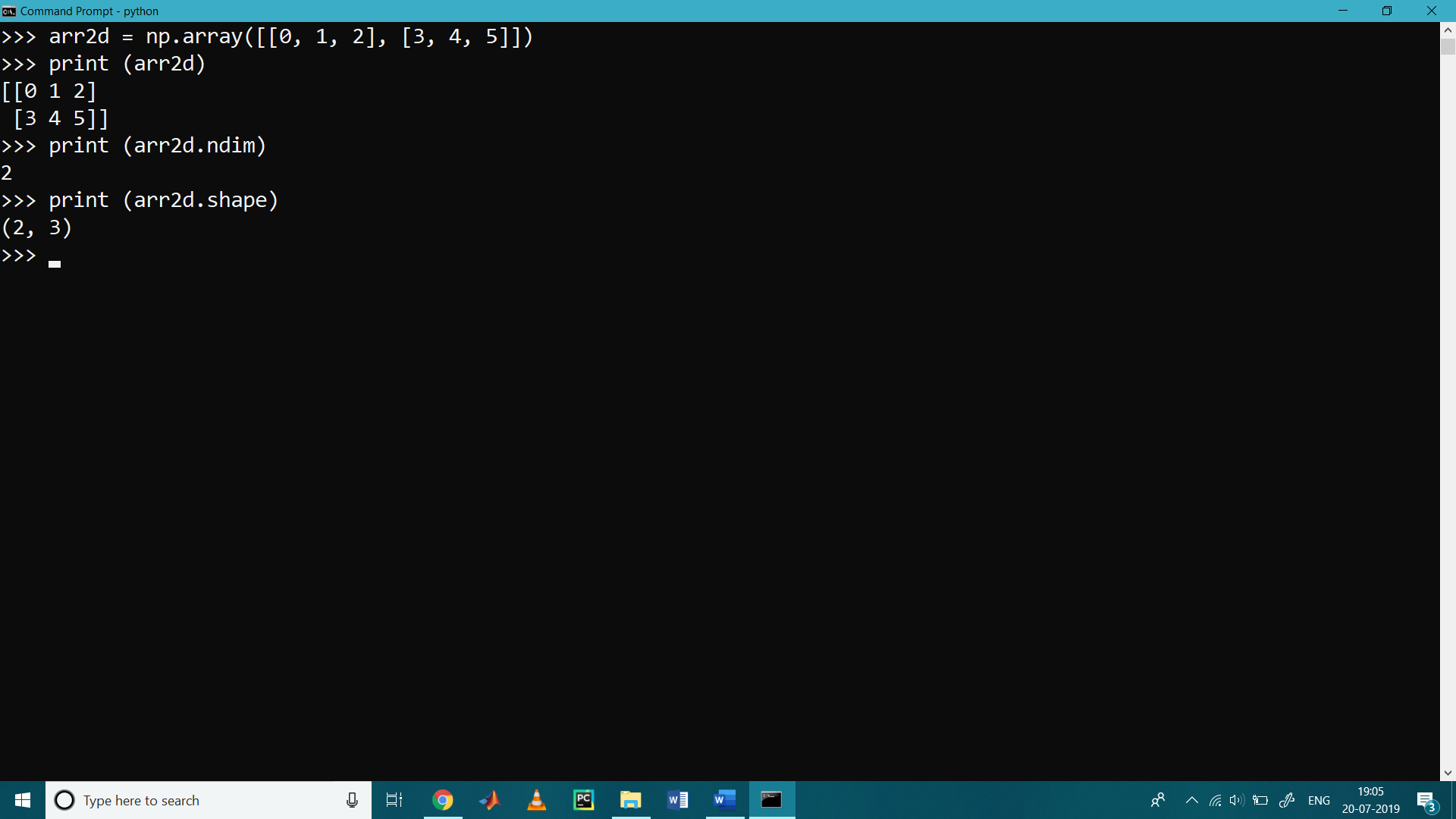Indexing with 2-D array

>>> arr2d = np.array([[0, 1, 2], [3, 4, 5]])

>>> print ( arr2d [0, 0] )

0

>>> print ( arr2d [0, 1] )

1

>>> print ( arr2d [1, 1] )

4

>>> print ( arr2d [1, 2] )

5

>>>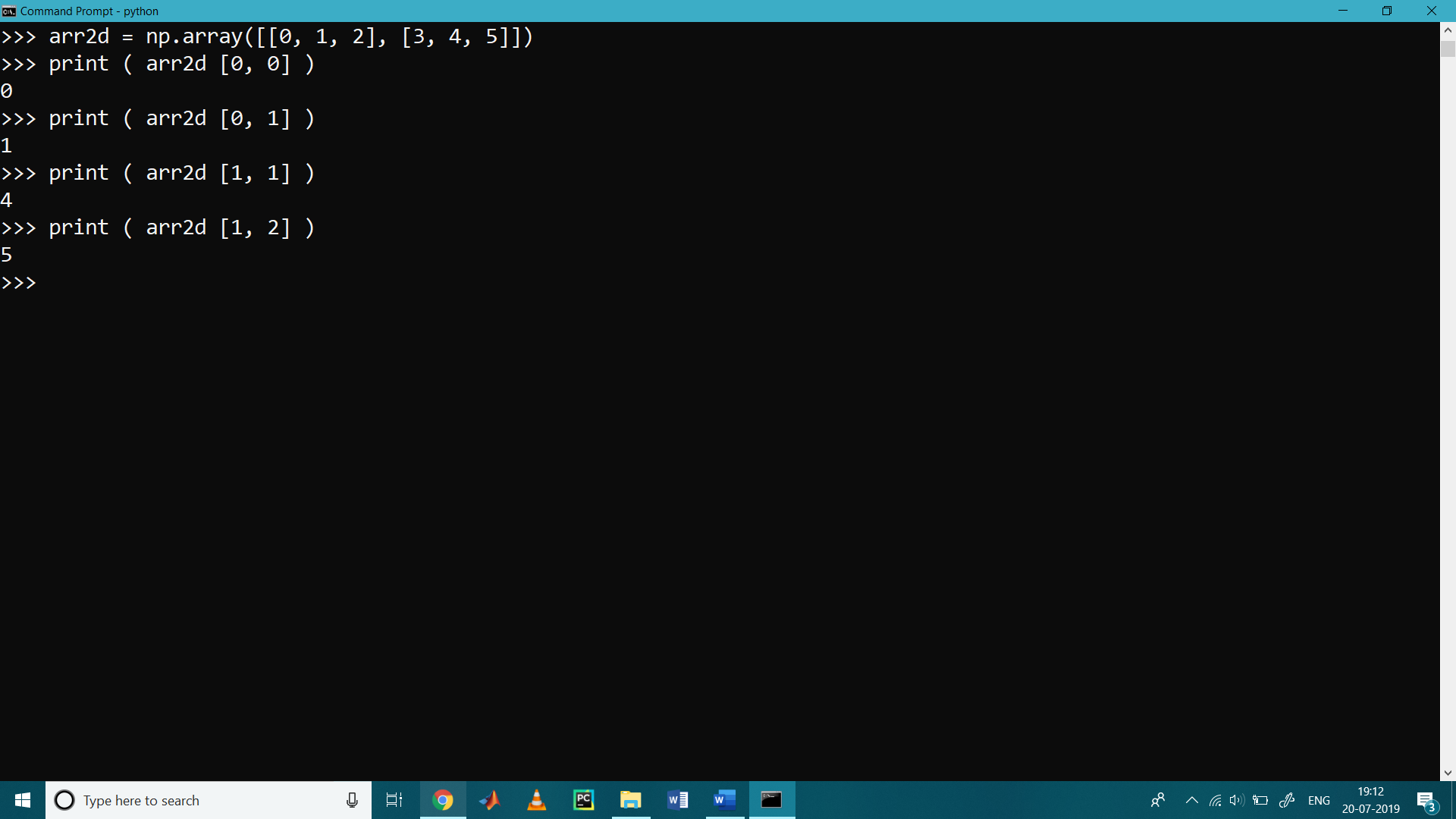Create an array of all zeros

>>> import numpy as np

>>> a = np.zeros ( ( 3 , 2 ) )

>>> print (a)

[[0. 0.]

[0. 0.]

[0. 0.]]

Create an array of all ones

>>> b = np.ones ( ( 3 , 2 ) )

>>> print ( b )

[[1. 1.]

[1. 1.]

[1. 1.]]

>>>

Create a constant array

>>> c = np.full( ( 3,3 ) , 9)

>>> print ( c )

[[9 9 9]

[9 9 9]

[9 9 9]]

Create a 2×2 identity matrix

>>> d = np.eye ( 3 )

>>> print ( d )

[[1. 0. 0.]

[0. 1. 0.]

[0. 0. 1.]]

Create an array filled with random values

>>> e = np.random.random((4,4))

>>> print ( e )

[[0.79463968 0.53404146 0.99421718 0.02112594]

[0.89371598 0.30352261 0.41096905 0.64765438]

[0.38907842 0.03502517 0.96261726 0.84007885]

[0.19656946 0.73345558 0.3992765  0.35990511]]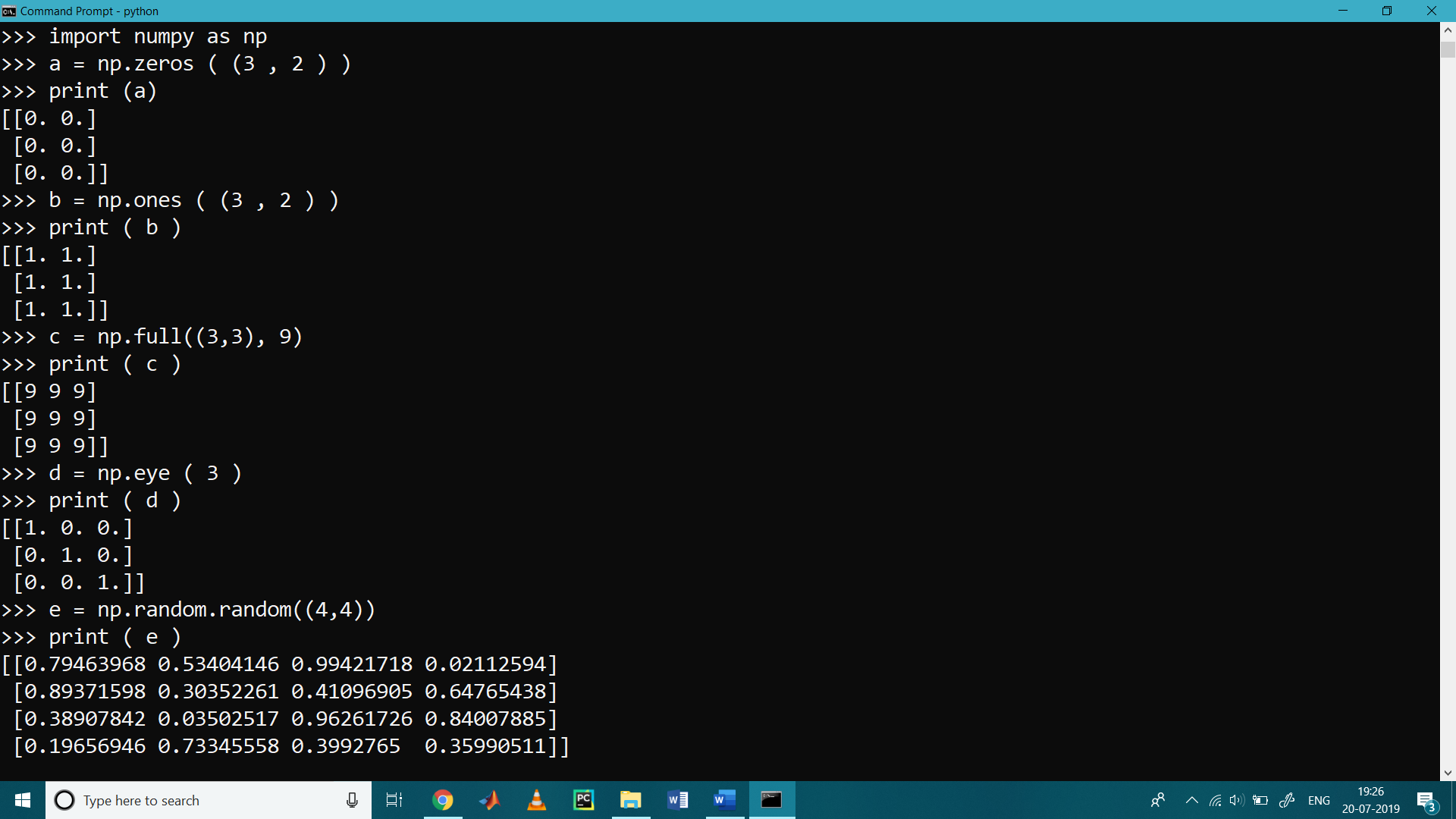Slicing

Like Python lists, numpy arrays also be sliced. Since arrays may be multidimensional, you must specify a slice for each dimension of the array:

#  create the array of size 3 by 4

>>> mult_arr = np.array ( [ [ 5, 6, 7, 10 ], [ 4, 3, 2, 1 ], [ 15, 12, 17, 20 ] ] )

Use slicing to pull out the subarray consisting of the first 2 rows and columns 1 and 2;

For that we create sub_arr having shape of (2, 2):

>>> sub_arr = mult_arr [:2, 1:3]

>>> print ( sub_arr )

[[6 7]

[3 2]]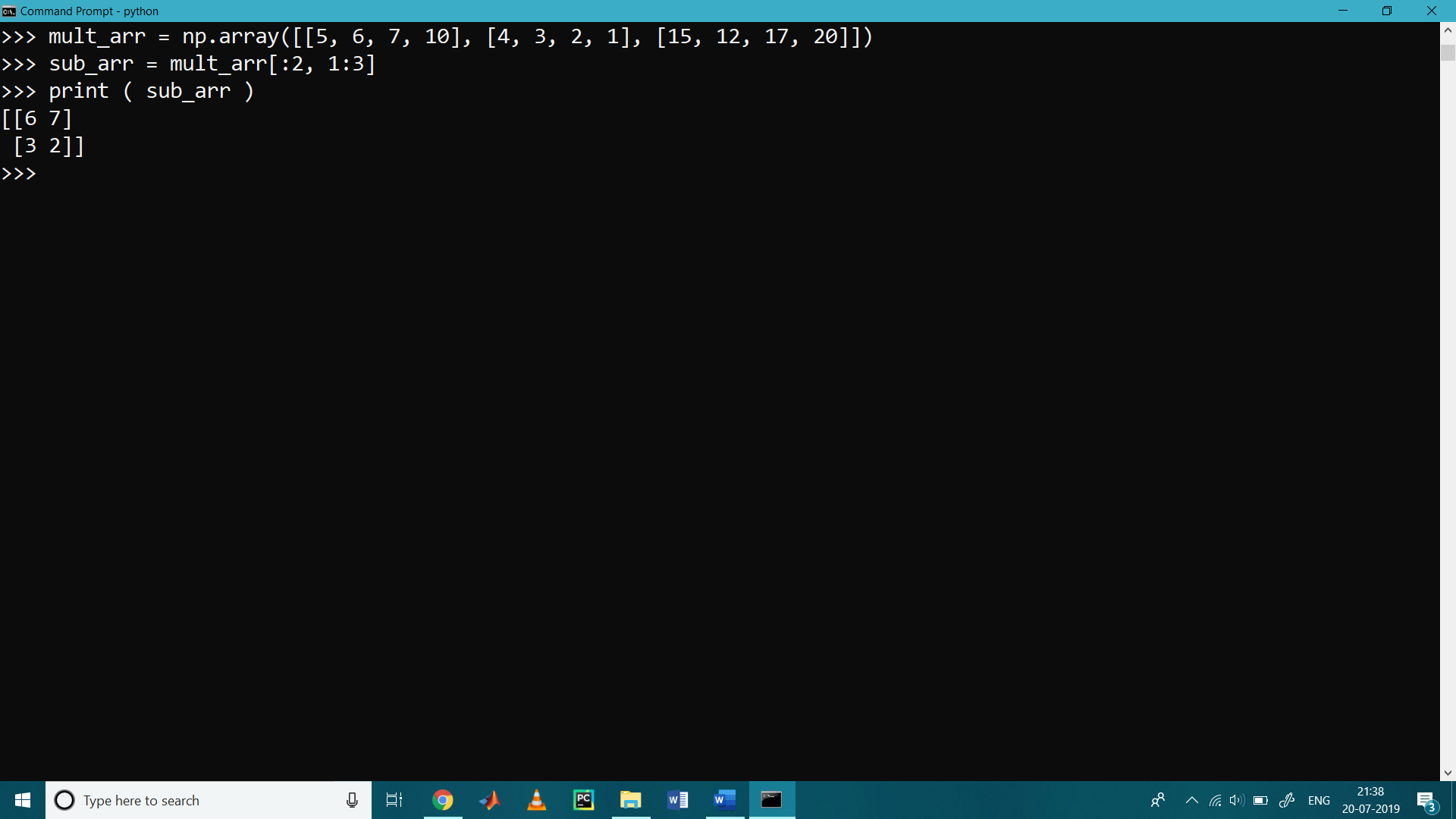Integer array indexing:

When you index into numpy arrays using slicing, the resulting array shall always be a subarray of the original array. In contrast, integer array indexing allows you to construct arbitrary arrays using the data from another array.

An example of integer array indexing is as follows,

>>> ar = np.array( [ [4, 5], [6, 7], [8, 9] ] )

>>> print ( ar [ [ 0, 1, 2], [ 0, 1, 0 ] ] )

[4 7 8]

The returned array will have shape (3,) and print [4 7 8]

The above example of integer array indexing is similar to the following line of code:

>>> print ( np.array ( [ ar [ 0, 0], ar [ 1, 1 ], ar [ 2, 0 ] ] ) )

[4 7 8]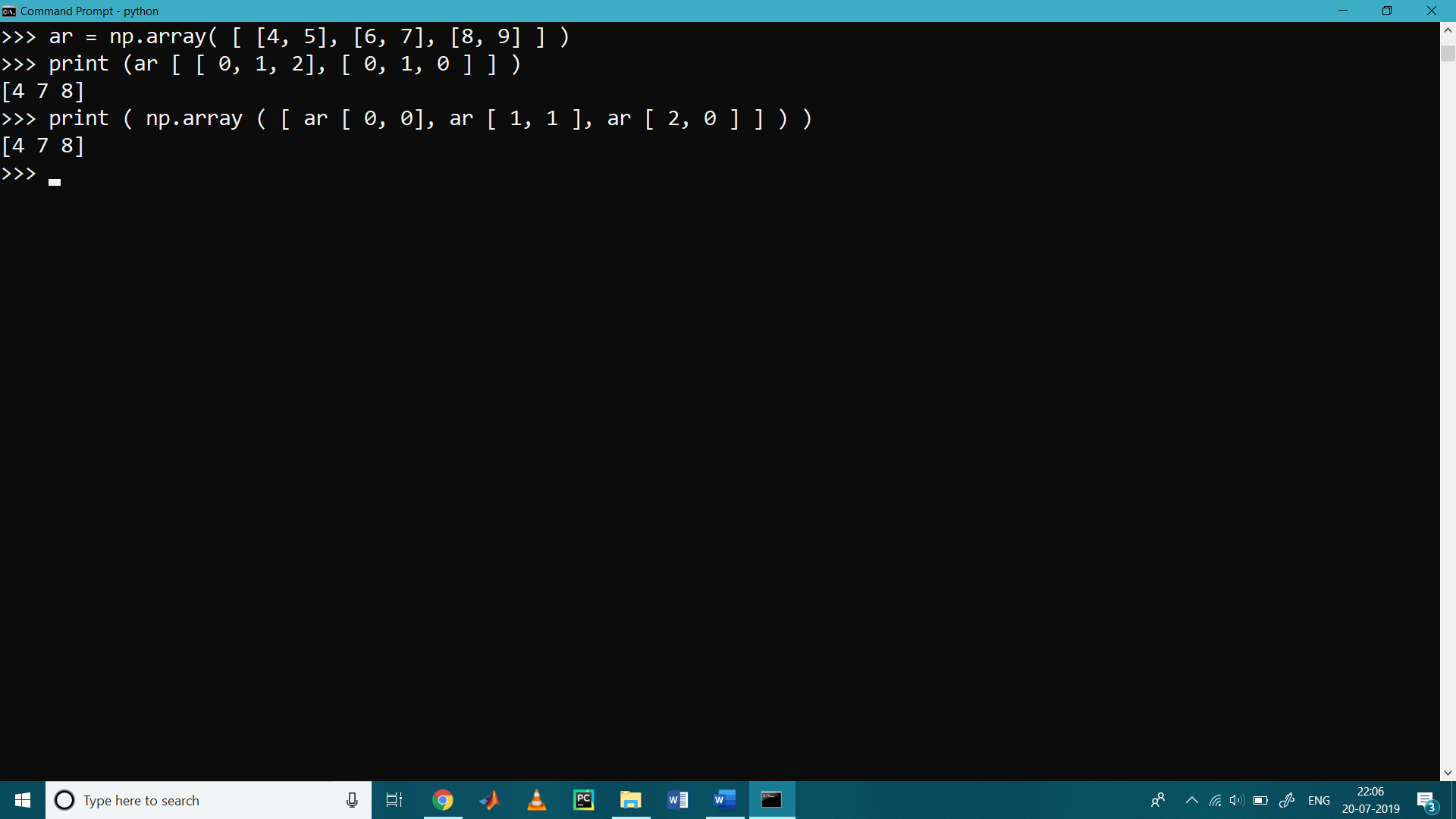Use of reshape function

It is used for resizing the array

>>> arr2 = np.array ( [ [8, 9, 10 ] , [ 1, 2, 3 ] ] )

>>> arr3 = arr2.reshape ( 3, 2 )

>>> print ( arr3 )

[[ 8  9]

[10  1]

[ 2  3]]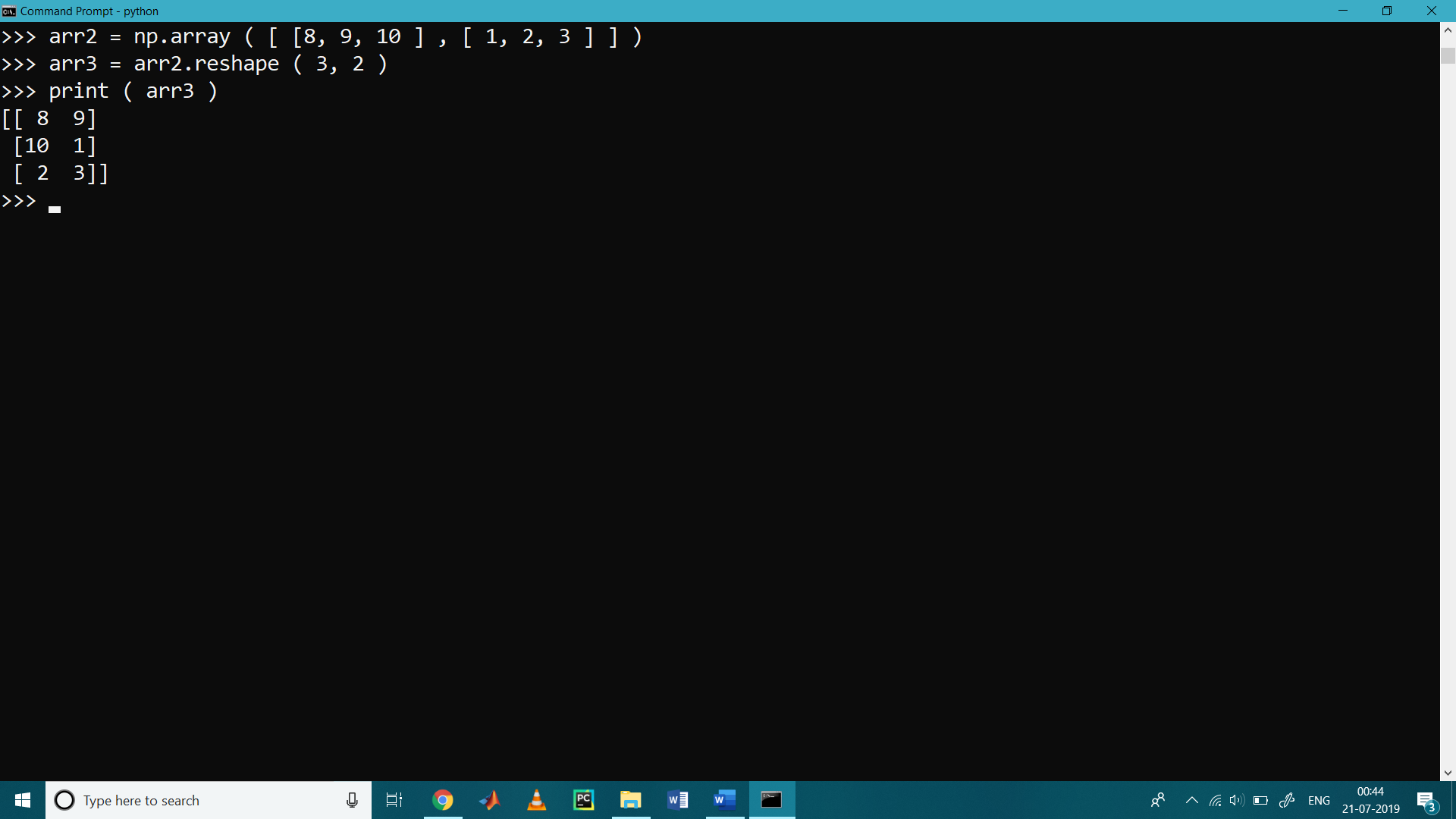NumPy Arange

The numpy.arange function returns a ndarray object containing evenly spaced values within the given range.  It returns an evenly spaced values within a given interval. For integer arguments, the method is equivalent to a Python built-in range function but returns the ndarray rather than a list.

The syntax of numpy.arange function is as follows,

numpy.arange (start, stop, step, dtype)

parameter are as follows,

start: it’s a number which is optional i.e, start of interval by default it is 0.

stop: it’s a number. End of interval

step : it’s a number which is optional.

dtype: The type of an output array.

>>> import numpy as np

>>> nparr = np.arange(5)  #stop=5

>>> print(nparr)

[0 1 2 3 4]

>>> nparr = np.arange( 2, 5 ) # start=2  stop 5

>>> print(nparr)

[2 3 4]

>>> nparr = np.arange( 2, 22, 2 ) # start=2 stop= 22 step=2

>>> print ( nparr )

[ 2  4  6  8 10 12 14 16 18 20]

>>> nparr = np.arange( 2, 22, 2, float ) #start=2 stop=22 step=2 and dtype=float

>>> print ( nparr )

[ 2.  4.  6.  8. 10. 12. 14. 16. 18. 20.]